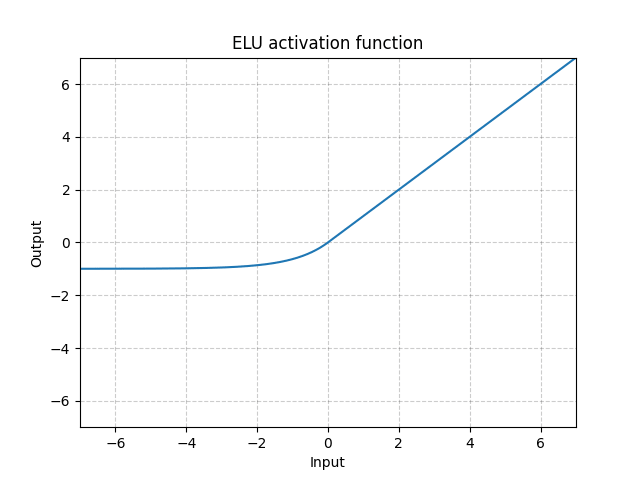/PyTorch

# ELU

class torch.nn.ELU(alpha: float = 1.0, inplace: bool = False) [source]

Applies the element-wise function:

$\text{ELU}(x) = \begin{cases} x, & \text{ if } x > 0\\ \alpha * (\exp(x) - 1), & \text{ if } x \leq 0 \end{cases}$
Parameters
• alpha – the $\alpha$ value for the ELU formulation. Default: 1.0
• inplace – can optionally do the operation in-place. Default: False
Shape:
• Input: $(N, *)$ where * means, any number of additional dimensions
• Output: $(N, *)$ , same shape as the inputExamples:

>>> m = nn.ELU()
>>> input = torch.randn(2)
>>> output = m(input)


© 2019 Torch Contributors
Licensed under the 3-clause BSD License.
https://pytorch.org/docs/1.7.0/generated/torch.nn.ELU.html